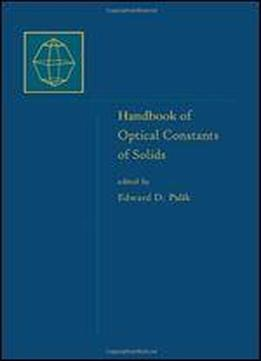# Handbook Of Optical Constants Of Solids: Handbook Of Thermo-optic Coefficients Of Optical Materials With Applications by Gorachand Ghosh / 1998 / English / PDF

This volume in the Handbook of Optical Constants of Solids is the first and only book to provide comprehensive coverage of refractive index and thermo-optic coefficients. It contains the refractive indexes and thermo-optic coefficients for nonlinear crystals, semiconductors, optical glasses, and fiber glasses. The Sellmeier coefficients of two physically meaningful Sellmeier equations are evaluated and are used to calculate these values throughout the normal transmission region of these optical materials. The author has also included analysis and explanations for some temperature-dependent optical devices/systems Presents the optical constants for over 70 technologically interesting crystals and commercial glasses Details various techniques for measuring the optical constants and mathematical models for analytical calculations of some data Includes thermo-optic coefficients that are necessary to calculate the refractive index of the optical material at any operating temperature Describes how all optical devices (both temperature dependent and independent) can be explained satisfactorily by using the two Sellmeier equations

views: 219